# HSSlive: Plus One & Plus Two Notes & Solutions for Kerala State Board

## BSEB Class 10 Maths Chapter 14 Statistics Ex 14.4 Textbook Solutions PDF: Download Bihar Board STD 10th Maths Chapter 14 Statistics Ex 14.4 Book AnswersBSEB Class 10 Maths Chapter 14 Statistics Ex 14.4 Textbook Solutions PDF: Download Bihar Board STD 10th Maths Chapter 14 Statistics Ex 14.4 Book Answers

BSEB Class 10th Maths Chapter 14 Statistics Ex 14.4 Textbooks Solutions and answers for students are now available in pdf format. Bihar Board Class 10th Maths Chapter 14 Statistics Ex 14.4 Book answers and solutions are one of the most important study materials for any student. The Bihar Board Class 10th Maths Chapter 14 Statistics Ex 14.4 books are published by the Bihar Board Publishers. These Bihar Board Class 10th Maths Chapter 14 Statistics Ex 14.4 textbooks are prepared by a group of expert faculty members. Students can download these BSEB STD 10th Maths Chapter 14 Statistics Ex 14.4 book solutions pdf online from this page.

## Bihar Board Class 10th Maths Chapter 14 Statistics Ex 14.4 Books Solutions

 Board BSEB Materials Textbook Solutions/Guide Format DOC/PDF Class 10th Subject Maths Chapter 14 Statistics Ex 14.4 Chapters All Provider Hsslive

## How to download Bihar Board Class 10th Maths Chapter 14 Statistics Ex 14.4 Textbook Solutions Answers PDF Online?

2. Click on the Bihar Board Class 10th Maths Chapter 14 Statistics Ex 14.4 Answers.
3. Look for your Bihar Board STD 10th Maths Chapter 14 Statistics Ex 14.4 Textbooks PDF.
4. Now download or read the Bihar Board Class 10th Maths Chapter 14 Statistics Ex 14.4 Textbook Solutions for PDF Free.

Find below the list of all BSEB Class 10th Maths Chapter 14 Statistics Ex 14.4 Textbook Solutions for PDF’s for you to download and prepare for the upcoming exams:

## BSEB Bihar Board Class 10th Maths Solutions Chapter 14 Statistics Ex 14.4

Question 1.
The following distribution gives the daily income of 50 workers of a factory :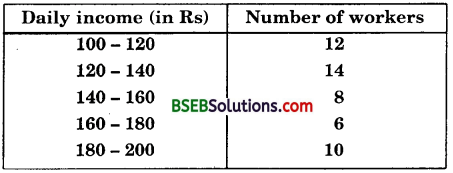Convert the distribution above to a less than type, cumulative frequency distribution and draw its ogive.
Solution:
Converting the given distribution to a less than type cumulative frequency distribution, we get:Let us now plot the points corresponding to the ordered pairs (120, 12), (140, 26), (160, 34), (180, 40) and (200, 50) on a graph.paper and join them by a free hand smooth curve.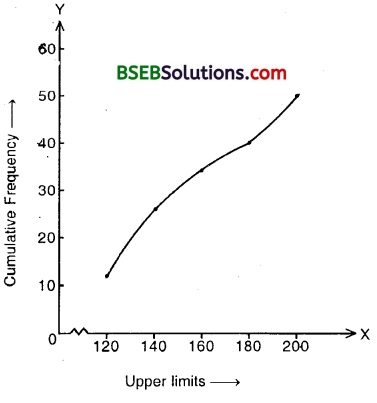The curve thus obtained is the required less than ogive.

Question 2.
During the medical check-up of 35 students of a class, their weights were recorded as follows :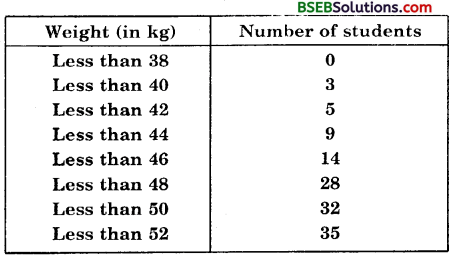Draw a less than type ogive for the given data. Hence obtain the median weight from the graph and verify the result by using the formula.
Solution:
Here the values 38, 40, 42, 44, 46, 48, 50 and 52 are the upper limits of the respective class-intervals. To represent the data in the table graphically, we mark the upper limits on the class intervals on x-axis and their corresponding cumulative frequencies on the y-axis, choosing a convenient scale.Let us now plot the points corresponding to the ordered pairs given by (38, 0), (40, 3), (42, 5), (44, 9), (46, 14), (48, 28), (50, 32) and (52, 35) on a graph paper and join them by a free hand smooth curve.
The curve thus obtained is the less than type ogive
To locate the median point on the graph, take 𝑛2 = 352 = 17.5 on the y-axis. From this point draw a line parallel to the x-axis cutting the curve at a. point. From this point, draw a perpendicular to the x-axis. The point of intersection of this perpendicular with the x-axis gives the median of the data. In this case it is 46.5.
Let us make the following table in order to find median by using the formula :Here, 352 , i.e., 17.5 will be in 46 – 48. Therefore 46 – 48 is the median class.
Here, l = 46, 𝑛2 = 352, cf = 14, f = 14
So, using the formula, median = l + (𝑛2−𝑐𝑓𝑓) x h
Median = 46 + (17.5−1414 x 2
= 46.5
Thus, median is verified.

Question 3.
The following tables gives production yield per hectare of wheat of 100 farms of a village :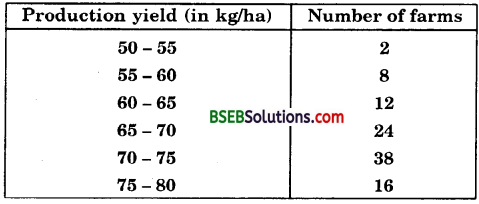Change the distribution to a more than type distribution, and draw its ogive.
Solution:
Converting the given distribution to a more than type distribution, we get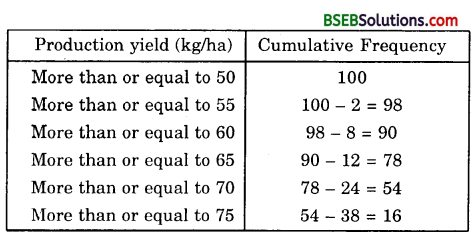Now, draw the ogive by plotting the points (50, 100), (55, 98), (60, 90), (65, 78), (70, 54) and (75, 16) on the graph paper and join them by a free hand smooth curve.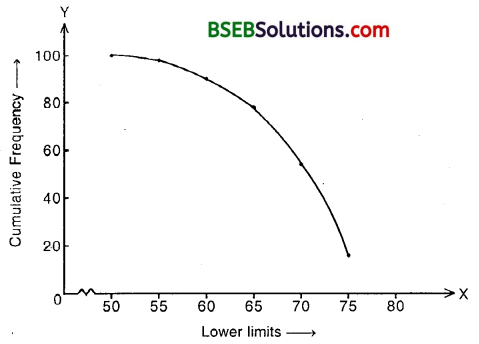The curve thus obtained is the required more than type ogive.

## Bihar Board Class 10th Maths Chapter 14 Statistics Ex 14.4 Textbooks for Exam Preparations

Bihar Board Class 10th Maths Chapter 14 Statistics Ex 14.4 Textbook Solutions can be of great help in your Bihar Board Class 10th Maths Chapter 14 Statistics Ex 14.4 exam preparation. The BSEB STD 10th Maths Chapter 14 Statistics Ex 14.4 Textbooks study material, used with the English medium textbooks, can help you complete the entire Class 10th Maths Chapter 14 Statistics Ex 14.4 Books State Board syllabus with maximum efficiency.

## FAQs Regarding Bihar Board Class 10th Maths Chapter 14 Statistics Ex 14.4 Textbook Solutions

#### Can we get a Bihar Board Book PDF for all Classes?

Yes you can get Bihar Board Text Book PDF for all classes using the links provided in the above article.

## Important Terms

Bihar Board Class 10th Maths Chapter 14 Statistics Ex 14.4, BSEB Class 10th Maths Chapter 14 Statistics Ex 14.4 Textbooks, Bihar Board Class 10th Maths Chapter 14 Statistics Ex 14.4, Bihar Board Class 10th Maths Chapter 14 Statistics Ex 14.4 Textbook solutions, BSEB Class 10th Maths Chapter 14 Statistics Ex 14.4 Textbooks Solutions, Bihar Board STD 10th Maths Chapter 14 Statistics Ex 14.4, BSEB STD 10th Maths Chapter 14 Statistics Ex 14.4 Textbooks, Bihar Board STD 10th Maths Chapter 14 Statistics Ex 14.4, Bihar Board STD 10th Maths Chapter 14 Statistics Ex 14.4 Textbook solutions, BSEB STD 10th Maths Chapter 14 Statistics Ex 14.4 Textbooks Solutions,
Share: